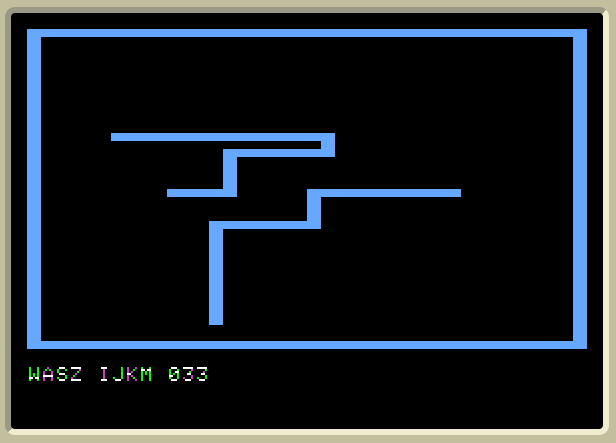SpeedWallerSpeedWaller is a two-line AppleSoft Basic program implementing a two-player graphics action game.

I wrote SpeedWaller many years ago (1989?), and upon recently finding it deep in the recesses of my filing cabinet, I decided that it was high time that I released it to the public. SpeedWaller was written in response to the numerous two-line AppleSoft Basic programming contests I used to see in Apple ][ magazines all the time such as Dr. Dobb's Journal. I wrote SpeedWaller over a period of many months in my high school computer lab during study hall, since there was little else to do. The last three months I remember distinctly because I was trying figure out how to shave off ten more characters in order to get it to fit in two lines. Crazy. :)

To the dismay of my future self, I was so exhausted with the game that when I was finally done I never submitted it to any of the contests, thereby missing out on endless fame, fortune and glory.

The program is, to the best of my knowledge, the only two-line two-player graphics action game in existence.
(Well, in Applesoft Basic, anyways, which has a 255 character limit. Sure, you could write a whole operating system in one line of C, it would just be a really long line)

You can download a disk image with speedwaller or you can run it directly on an Apple ][ emulator in your browser courtesy Apple][js. Just type "RUN SPEEDWALLER"

OLD (sites are now gone):
I have since discovered it archived at: openix The maintainer says ``Never before has so much been done with so little.'' Also: UIowa, Phandel

If you try it out, please tell me what you think, via email, at:

Typing In SpeedWaller

This program must be typed in exactly as shown, WITHOUT spaces, on an Apple II computer (or emulator).

For example:

]1ONSCRN(X,Y)<>7ANDB<>0ANDSCRN(U,V)  etc...

Running SpeedWaller

Keys to play the game:
Player 1                Player 2

W                       I

^                       ^
|                       |
A <-+-> S               J <-+-> K
|                       |
v                       v

Z                       M
Try playing the game before you read the rules in the "Hints" file.

The Program

(Okay - it's three lines - but the REM comment doesn't count. :)

0 REM SPEEDWALLER - COPYRIGHT DAVID LJUNG - I RETAIN ALL RIGHTS AND
OWNERSHIP OF THIS SOFTWARE - TO CHANGE AT MY DISCRETION - THIS REM
MUST REMAIN WITH THE PROGRAM UNCHANGED.  CREATED 1988-1989 BY
DAVID LJUNG - EMAIL SPEEDWALLER@DAVESOURCE.COM

1 ONSCRN(X,Y)<>7 AND B<>0 AND SCRN(U,V) <> 7 GOTO 2: HOME : GR :
X=10 : Y=20 : U=30 : V=Y : I=201 : J=202 : K=203 : M=205 : W=215 :
A=193 : S=211 : Z=218 : O=1 : Q=-1 : P=49152 : COLOR=7 : HLIN 0,39
AT 0 : HLIN 0,39 AT 39 : VLIN 0,39 AT 0 : VLIN 0,39 AT 39 : VTAB 22 :
? "WASZ IJKM  "C : ON B=0 GOTO 2 : CALL -678 : RUN
2 PLOT X,Y : PLOT U,V : B=PEEK(P) : G= B<>Z AND B<>W: H=B<>A AND B<>S :
O=G*(O*H+(B=S)-(B=A)) : L=H*(L*G+(B=Z)-(B=W)) : G=B<>I AND B<>M :
H=B<>J AND B<>K : Q=G*(Q*H+(B=K)-(B=J)) : R=H*(R*G+(B=M)-(B=I)) :
X=X+O : Y=Y+L : U=U+Q : V=V+R : FOR N=1 TO 99 : NEXT : C=C+1 :
VTAB 22 : HTAB 12 : ? C : GOTO 1
Type it in like this mess:
1ONSCRN(X,Y)<>7ANDB<>0ANDSCRN(U,V)<>7GOTO2:HOME:GR:X=10:Y=20:U=30:V=Y:I=201:J=202:K=203:M=205:W=215:A=193:S=211:Z=218:O=1:Q=-1:P=49152:COLOR=7:HLIN0,39AT0:HLIN0,39AT39:VLIN0,39AT0:VLIN0,39AT39:VTAB22:?"WASZ IJKM  "C:ONB=0GOTO2:CALL-678:RUN
2PLOTX,Y:PLOTU,V:B=PEEK(P):G=B<>ZANDB<>W:H=B<>AANDB<>S:O=G*(O*H+(B=S)-(B=A)):L=H*(L*G+(B=Z)-(B=W)):G=B<>IANDB<>M:H=B<>JANDB<>K:Q=G*(Q*H+(B=K)-(B=J)):R=H*(R*G+(B=M)-(B=I)):X=X+O:Y=Y+L:U=U+Q:V=V+R:FORN=1TO99:NEXT:C=C+1:VTAB22:HTAB12:?C:GOTO1
Total characters: 239+239 = 478 (16 characters are for "instructions" and the counter)

I retain all rights and ownership to this 'software'. Redistribution without fee is permitted so long as my name remains and the original, unmodified code is present. I also retain the right to give ownership of this software away at my discretion.

David Ljung

SpeedWaller-at-Daveola.com
Version 1.00
Created April, 1989
November, 1994
Updated 9/1999